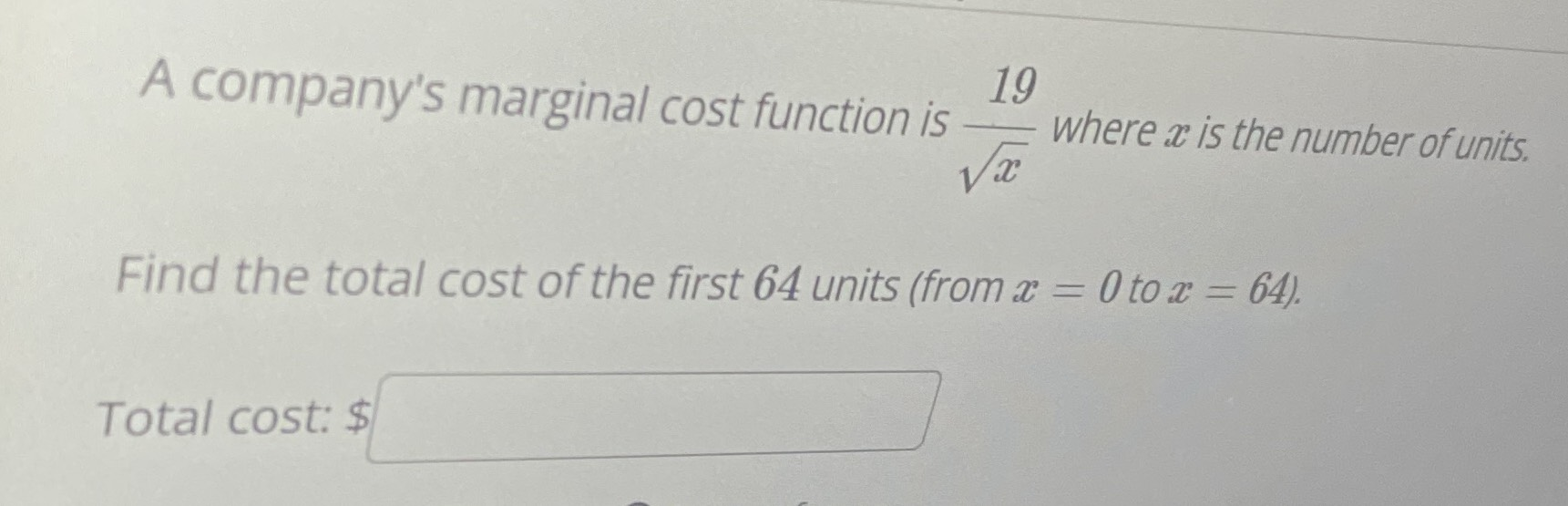### ¿Todavía tienes preguntas de matemáticas?

Pregunte a nuestros tutores expertos
Algebra
PreguntaA company's marginal cost function is $$\frac { 19 } { \sqrt { x } }$$ where $$x$$ is the number of units. Find the total cost of the first $$64$$ units (from $$x = 0$$ to $$x = 64$$ ). Total cost: $$\ \square$$